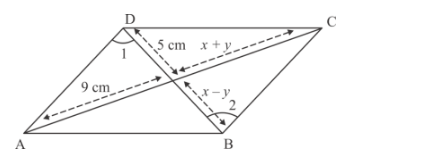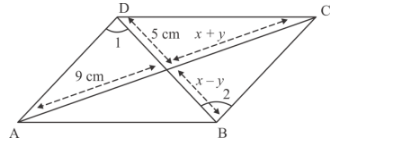# In Figure 2, ABCD is a parallelogram.`
Question:

In Figure 2, ABCD is a parallelogram. Find the values of x and y.Solution:

The given parallelogram isWe have to find the value of x and y.

We know that if two diagonals are drawn in a parallelogram, then the intersection points is the mid-point of the two diagonals. Therefore, we have two equations as follow

x + y = 9…… (1)

x − y = 5…… (2)

Now, add the equation (1) and equation (2), we get

$2 x=14$

$\Rightarrow \quad x=7$

Now, we are going to put the value of x in equation (1), we get

$7+y=9$

$\Rightarrow y=2$

Hence the value of $x$ and $y$ are $x=7$ and $y=2$